# Curve Wizard PropertyManager

The Curve Wizard creates boundary and section curves in point cloud and mesh files.

You can also import and edit .ibl, .igs, .txt, and .csv files containing point data that defines discrete curve sets. The curves are created in a 3D sketch and are fully editable in the SOLIDWORKS application. You can create surfaces from these curves, using features such as lofts and boundary surfaces.

## Mesh/Cloud/File

Select a mesh, sub-mesh, or point cloud. If you select Discrete under Creation Method, click Browse and select an .ibl, .igs, .txt, or .csv file.

## Creation Method

 Discrete Creates a discrete curve set from a file you import. Select an .ibl, .igs, .txt, or .csv file.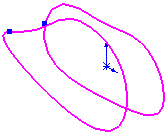Section Curve Creates section curves from the intersection of a series of planes and a mesh or point cloud.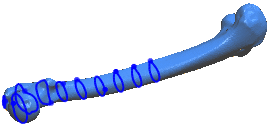Boundary Curve Creates a curve along the mesh boundary. A boundary forms along boundary edges. ScanTo3D can create boundary curves only along boundary edges. You can smooth the mesh boundary with the Smoothing PropertyManager in the Mesh Prep Wizard. Video: Smoothing a Mesh Boundary.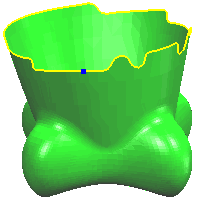## Section Plane Parameters (Section Curves Only)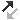Section Plane Select a pair of points, a plane, or an existing curve (so you can create section curves perpendicular to it). Click Reverse Direction , if necessary, to switch the direction in which the section curves are created. Two points selected for Section Plane define a vector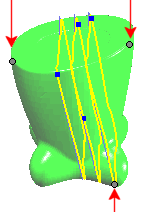Selected Point on Start Plane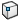Selecting a pair of points defines a vector. The software creates intermediate section planes perpendicular to the vector starting from the selected Point on Start Plane.Point on Start Plane Select a mesh vertex, sketch point on the mesh, curve vertex, or model vertex.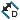Plane Distance Sets the distance between section planes.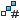Intermediate Section Planes

## Creation Parameters

You must first select a curve in Editing Curve, then adjust the Curve Fit or modify the curve's shape using Edit Tools.

Curve Fit

 Curve Fit Adjusts curve fitting tolerance. Move the slider to the left to sample more vertices or points, making the curve fit more tightly to the mesh or cloud. Move it to the right to loosen the curve fit. Video: Adjusting the Curve Fit Tolerance The example shows the curve loosening as you move the slider from left to right. Editing Curve Lists the section or boundary curves. Select a curve from the list, then adjust the Curve Fit or modify the curve's shape using Edit Tools.

Edit Tools

Points and vertices you select are projected back to the curve's plane. Example using Add Point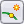: Video: Projecting Points to the Curve's Plane.Add Point (Section curves only.) Adds points that refine the curve position. Select mesh vertices to add curve-defining points.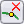Delete Point Deletes points used to create the curve. You can create a curve which more accurately represents the designer's intent by removing points or vertices related to noise in the scanned data. Select a curve in Editing Curve, click Delete Point, then select points to delete that were created on the mesh vertices to refine the curve. Deleting points changes the curve's shape.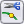Break Curve Adds and displays break points on the curve where two curves connect. You can set the connectivity at break points to be Contact, Tangent, or Smooth (curvature continuous). Video: Changing Break Points on a Curve Example shows break point changing from Contact, to Tangent, to Smooth.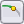Trim Curve (Section curves only). Trims the selected curve.